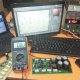Breaking News
Home / Amplifier Diagram / Transistor circuit diagram of 2sc5200 and 2sa1943

# Transistor circuit diagram of 2sc5200 and 2sa1943

## Transistor circuit diagram of 2sc5200 and 2sa1943:

Here in this article, we can learn how to make a transistor circuit diagram of 2sc5200 and 2sa1943. We can use 4 transistors in this circuit. 2 pieces of 2sa1943 and 2 pieces of 2sc5200. How many voltages and amperes have to use? We know one transistor can take maximum 1.2 amperes so here in this circuit, we can use 4 transistors. We know Voltage X Ampere = Watts. we can use 4 transistors so 1.2X4=4.8. So we can use 4.8 amperes in this circuit. How many Watts can we get from this circuit? We can use 4.8 amperes. If we use 40 Voltage and 5-ampere transformer then we can get Voltage X ampere= Watts, So 40X5=200 Watts. Please remember that Always use original or good quality one transistor. How to know about the original transistor?

this is the circuit diagram.there have many groups of the transistor in our market. For an example, 2sa5200 is the transistor number, and there also have another number, that is the Group number it starts from 120 to up 900++. Please always try to use up to 500++ Group number of the transistor.Center tap transformer for transistor amplifier. transforms is a electrical energy from one circuit to another without any direct electrical connection. We had to use the transformer everywhere. but nowadays we can use SMPS circuit also. in SMPS also have the center tap. Normally we use Positive and Negative voltage transformer. but center tap has 3 output. Positive, Negative and Ground Voltage. we need this transformer for making the amplifier. normally in a transformer has primary and the secondary wire. for primary we use AC 110v/220 voltage. and we can get output from secondary what’s we need. here secondary has 2 wire output. But in a center tap transformer has 3 wire for the output voltage. For this circuit, we have to use center tap transformer. please share this post if you are like.

transistor circuit diagram of 2sa1943 and 2sc5200

Thanks a lot to be with us. If you want another post then please visit our website.

We have another post for you. like as repairing amplifier,